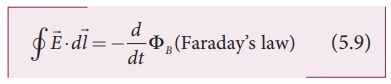Home | | Physics 12th Std | MaxwellŌĆÖs equations in integral form

# MaxwellŌĆÖs equations in integral form

Electrodynamics can be summarized into four basic equations, known as MaxwellŌĆÖs equations.

MaxwellŌĆÖs equations in integral form

Electrodynamics can be summarized into four basic equations, known as MaxwellŌĆÖs equations. These equations are analogous to NewtonŌĆÖs equations in mechanics. MaxwellŌĆÖs equations completely explain the behaviour of charges, currents and properties of electric and magnetic fields. These equations can be written in integral form (or integration form) or derivative form (or differentiation form). The differential form of MaxwellŌĆÖs equation is beyond higher secondary level because we need to learn additional mathematical operations like curl of vector fields and divergence of vector fields. So we focus here only in integral form of MaxwellŌĆÖs equations:

1. First equation is nothing but the GaussŌĆÖs law. It relates the net electric flux to net electric charge enclosed in a surface. Mathematically, it is expressed aswhere E is the electric field and Qenclosed is the charge enclosed. This equation is true for both discrete or continuous distribution of charges. It also indicates that the electric field lines start from positive charge and terminate at negative charge. This implies that the electric field lines do not form a continuous closed path. In other words, it means that isolated positive charge or negative charge can exist.

2. Second equation has no name. But this law is similar to GaussŌĆÖs law in electrostatics. So this law can also be called as GaussŌĆÖs law in magnetism. The surface integral of magnetic field over a closed surface is zero. Mathematically,whereis the magnetic field. This equation implies that the magnetic lines of force form a continuous closed path. In other words, it means that no isolated magnetic monopole exists.

3. Third equation is FaradayŌĆÖs law of electromagnetic induction. This law relates electric field with the changing magnetic flux which is mathematically written aswhereis the electric field. This equation implies that the line integral of the electric field around any closed path is equal to the rate of change of magnetic flux through the closed path bounded by the surface. Our modern technological revolution is due to FaradayŌĆÖs laws of electromagnetic induction. The electrical energy supplied to our houses from electricity board by using FaradayŌĆÖs law of induction.

4. Fourth equation is modified AmpereŌĆÖs circuital law. This is also known as Ampere ŌĆō MaxwellŌĆÖs law. This law relates the magnetic field around any closed path to the conduction current and displacement current through that path.whereis the magnetic field. This equation shows that both conduction and also displacement current produces magnetic field. These four equations are known as MaxwellŌĆÖs equations in electrodynamics. This equation ensures the existence of electromagnetic waves. The entire communication system in the world depends on electromagnetic waves. In fact our understanding of stars, galaxy, planets etc come by analysing the electromagnetic waves emitted by these astronomical objects.

Tags : Electromagnetic Waves , 12th Physics : Electromagnetic Waves
Study Material, Lecturing Notes, Assignment, Reference, Wiki description explanation, brief detail
12th Physics : Electromagnetic Waves : MaxwellŌĆÖs equations in integral form | Electromagnetic Waves